# Design of Storm Water Drains with Excel Spreadsheets using a Weir or Orifice Equation

## Introduction

In the design of storm water drains, the openings used to drain storm water runoff into the storm sewer system may be a curb inlet, gutter inlet, or a combination of the two. A major part of the design procedure for storm drains is to use the design storm water runoff rate to size the surface openings and/or curb inlets so that they can handle the storm water runoff. In the design of stormwater drains, the stormwater curb inlet or gutter inlet opening can be sized using orifice equations if the opening is completely submerged, or with a weir equation if the inlet opening is not submerged.

The first thing needed for sizing curb inlets is the storm water runoff rate for a design storm. The design storm water flow for the inlet is typically calculated using the rational method, and would be the same runoff rate used for hydraulic design of the storm sewer being fed from the inlet. Continue with this article for storm water inlet design equations, example calculations, and Excel spreadsheets that can be downloaded to make the calculations.

## Types of Pavement Drain Inlets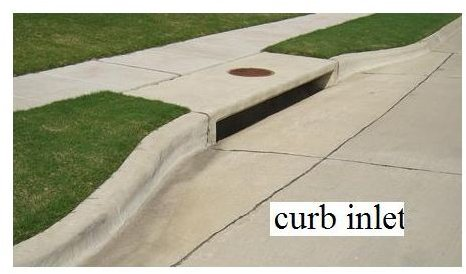Several types of pavement inlets are in common use in the design of storm water drains. Such storm water inlets are needed for draining stormwater runoff into a storm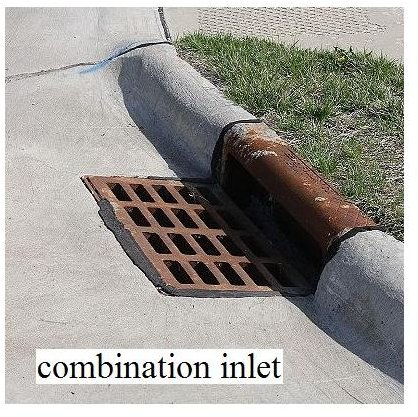sewer. The main types of storm drains are the curb inlet, gutter inlet, and combination inlet (with both a curb opening and gutter opening). Also, each type of inlet may be either depressed or undepressed. The picture at the left shows a curb inlet and the picture at the right shows a combination inlet.

Combination Inlet Image Credit: Wikimedia Commons

Curb Inlet Image Credit: Lone Star Manhole and Structures

A gutter inlet would have the grate in the gutter, like the combination inlet, but no curb opening. Both of the inlets shown in the pictures are undepressed, that is the gutter isn’t depressed at the inlet.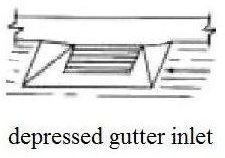The depressed gutter inlet shown in the figure at the left on the bottom uses a depression in the gutter at the opening to aid in capturing the stormwater flowing over the inlet. A combination inlet or a curb inlet can also be designed with a depression at the inlet. Gutter inlets will almost always have grates, but a curb inlet is usually open without any grates, as you can see in the pictures. As shown in the next two sections, the process for design of stormwater drains can use either an orifice equation or a weir equation to determine the required size for the curb and/or gutter opening.

Depressed Gutter Inlet Image Credit: H. Bengtson

Go on to page 2 for discussion of storm water inlet design equations and parameters using an orifice equation and using a weir equation. There is also an example calculation and there are Excel spreadsheets that can be downloaded from page 3.

## Use of an Orifice Equation for Inlet Sizing

A grated gutter inlet that is fully submerged at the design storm water flow, or a curb inlet that is fully submerged at design flow, can be sized using the orifice equation: Q = Co A√(2gde), where:

Q is the design storm water runoff rate that must pass through the opening, cfs, (m3/s for S.I. units)

A is the open area of the inlet, ft2, (m2 for S.I. units)

de is the height of storm water above the centroid of the opening, ft. (m for S.I. units)

NOTE: de = d – h/2, for a curb opening, where d is the depth of storm water above the bottom of the opening.

g is the acceleration due to gravity, 32.2 ft/sec2, (9.82 m/s2 for S.I unitsd

Co is the orifice coefficient (dimensionless). A value of 0.67 is often used for storm water inlet design.

NOTE: The orifice equation [ Q = CoA√(2gd) ] is the same for either U.S. or S.I. units.

## Use of a Weir Model for Inlet Sizing

A curb inlet that isn’t submerged at design storm water flow, or a gutter inlet that isn’t submerged, can be sized using the horizontal, sharp-crested weir equation: Q = CwLd1.5, where

Q is the design storm water runoff rate that must pass through the inlet, cfs, (m3/s for S.I. units)

L is the length of the curb inlet opening (or the length of the gutter opening over which storm water flows), ft, (m for S.I. units)

d is the depth of storm water above the bottom of the curb inlet opening (or above the gutter inlet opening), ft, (m for S.I. units)

Cw is the weir coefficient, a dimensionless constant that ranges from 2.3 – 3.3 for U.S. units. (1.27 – 1.8 for S.I. units)

NOTE: Use of Cw = 2.3 for U.S. units or 1.27 for S.I. units is recommended in references #2 and #3 below. This gives a conservative value for the gutter or curb opening length.

## Example Calculation

Sizing a curb inlet for storm water runoff is shown in the following examples illustrating design of storm drains.

Problem Statement #1: Determine the length of curb inlet opening required to drain storm water runoff of 2.4 cfs at a depth of 5 inches, through a curb opening with a height of 6 inches, using a weir discharge coefficient value of 2.3.

Solution: Substituting Q = 2.4 cfs, Cw = 2.3, and h = 5/12 ft into the weir equation gives: 2.4 = (2.3)L[(5/12)1.5]. Solving this equation for L gives: L = 3.9 ft. = curb inlet opening length.

Problem Statement #2: Determine the length of curb inlet opening required to drain storm water runoff of 2.4 cfs at a depth of 5 inches through a curb opening with a height of 4 inches, using an orifice coefficient of 0.67.

Solution: Substituting d = 5/12 ft and h = 4/12 ft into de = d – h/2, gives de = 3/12 = 0.25 ft. Then substituting Q = 2.4 cfs, Co = 0.67, h = 4/12 ft, and de = 0.25 ft, into Q = Co h L (2g de)1/2, and solving for L, gives: L = 2.7 ft = curb inlet opening length.

Go to page 3 for presentations of Excel spreadsheets to make this type of calculations and links to download the spreadsheets.

## Excel Spreadsheets for Storm Water Curb Inlet Design

The Orifice Equation for a submerged curb inlet: The image at the left shows an Excel spreadsheet that is set up for curb inlet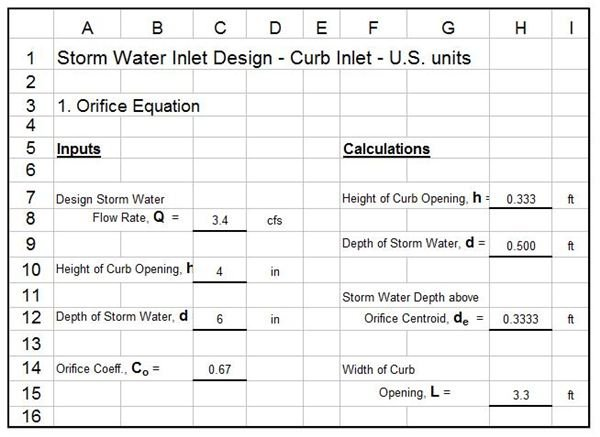design with the orifice equation. The image at the right shows a cross-sectional view of a submerged curb outlet,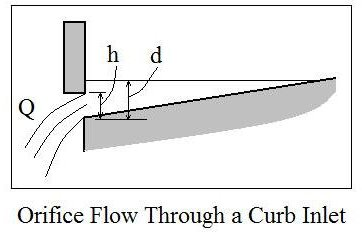for which this spreadsheet can be used.

The orifice equation for a submerged curb inlet is: Q = Co h L (2g de)1/2, where: de = d – h/2. Note that the design storm water runoff rate, Q; the height of the curb opening, h; and the storm water depth at the curb opening, d, are shown in the diagram. They are also defined in the previous sections, along with the other parameters in the equation, Co, L, g, and de.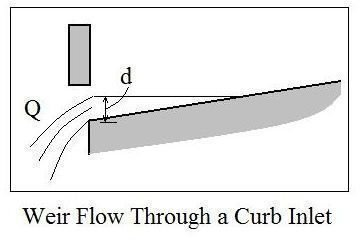The Weir Equation for a curb inlet that isn’t submerged: The image at the left shows a diagram of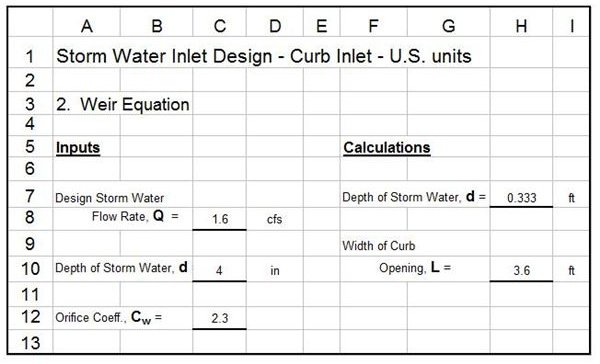storm water flow through an unsubmerged curb outlet. The image at the right is an Excel spreadsheet for curb inlet design with the weir equation, which for unsubmerged flow through a curb inlet becomes: Q = Cw L d1.5. The parameters, Q and d are shown in the diagram, while Cw and L are defined in a previous section.

Excel spreadsheets for design of curb inlets using either the orifice equation or the weir equation with either U.S. units or S.I. units can be downloaded by clicking on one of the links below.

## Excel Spreadsheets for Storm Water Gutter Inlet Design

The orifice equation for a submerged gutter inlet: The image at the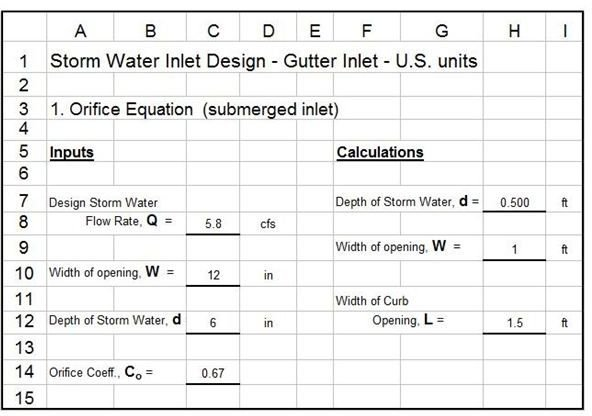left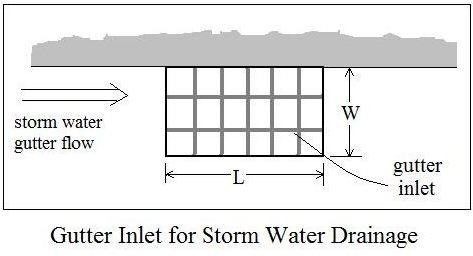shows a plan view of a grated gutter inlet. The image at the right shows an Excel spreadsheet to size a gutter inlet that is submerged, using the orifice equation. For this application, the orifice equation is: Q = Co(WL)(2gd)1/2, where Q, the design storm water runoff rate, d, the storm water depth, and g are as defined above. The storm water inlet width and length, W and L, are shown in the figure at the left.

The weir equation for a nonsubmerged gutter inlet: The image at the left shows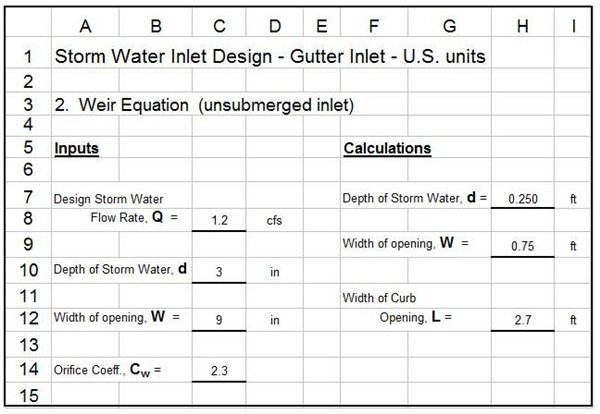an Excel spreadsheet to size a gutter inlet that is not submerged, using the weir equation. For a nonsubmerged gutter inlet, the weir equation becomes: Q = Cw(L + 2W)d1.5, where Q, L, W, and d are the same as used in the orifice equation above. Cw is the weir coefficient. Typical values used for Cw are 2.3 for U.S. units and 1.27 for S.I. units.

To download Excel spreadsheets for both the orifice equation and the weir equation in either U.S. or S.I. units, click on one of the links below.

## References

References for Further Information:

1. McCuen, Richard H., Hydrologic Analysis and Design, 2nd Ed, Upper Saddle River, NJ, 1998.

2. ASCE. 1992. Design and Construction of Urban Stormwater Management Systems. The Urban Water Resources Research Council of the American Society of Civil Engineers (ASCE) and the Water Environment Federation. American Society of Civil Engineers, New York, NY.

3. Texas Department of Transportation/Online Hydraulic Design Manual/Storm Drain Inlets